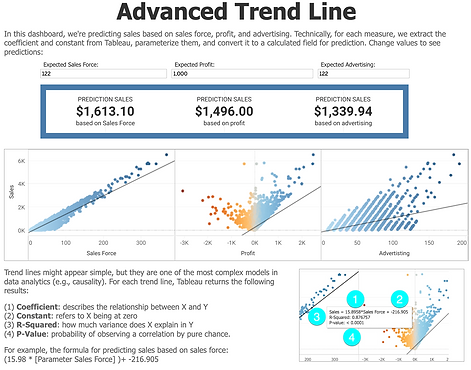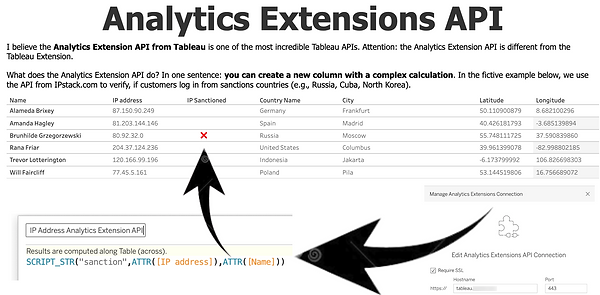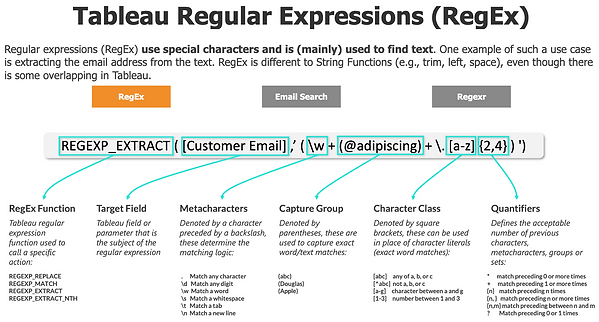top of page

With a deep understanding of trend lines (i.e., linear regression), we can empower the user to predict business scenarios:

(1) Coefficient: describes the relationship between X and Y

(2) Constant: refers to X being at zero

(3) R-Squared: how much variance does X explain in Y

(4) P-Value: the probability of observing a correlation by pure chance.

For example, the formula for predicting sales based on sales force:

(15.98 * [Parameter Sales Force] )+ -216.905For the Root Cause Dashboard, we borrow a popular technique from Machine Learning: FEATURE SELECTION. In statistical modeling, feature selection is the process of choosing features that are most useful for predictors. In our case, we want to know the most dominant features causing delays.

A good forecasting model captures the way in which things are changing. In general, there are four ways an environment changes:

(1) SEASONALITY

(2) TREND

(3) SEASONALITY AND TREND

(4) RANDOMNESS

In short, forecasting is really hard.Tableau is not a programming language such as R or Python where we can test a hypothesis. However, we can use Tableau to visualize and communicate the results of a hypothesis test.

In Tableau, we can "train" our clustering algorithm on historical data (image left) and then use the very same algorithm (as a group) to predict the profitability of new customers.Set theory is a branch of mathematical logic that studies set, which informally are a collection of objects often used in discrete mathematics. In Tableau, we have:

- Fixed sets
- Dynamic sets

- Combined sets

"Fixed sets" are hardly ever used as they are hardcoded members. "Dynamic sets" are most commonly used with top-N or in combination with parameters.

Pretty cool that we can visualize one of the most important mathematical concepts directly in Tableau.Even though Tableau is not a programming language, with a bit of creativity, we can add some complex algorithms natively into Tableau.What's the probability that out of 50 people, at least two have the same birthdate?

My old-time favorite algorithm: Monte Carlo simulations.As a Swiss person, I have to give credit to one of our most famous mathematicians: Jacob Bernoulli.Tableau's Analytics Extension API is easily the most underrated API.Extracting patterns from the day of the week.One of my earliest dashboards around 2018.So important ...Coded directly with Python - probably simpler with the Analytics Extension API.If we really want to drill down in maps, we need latitude and longitude ...

Great formula.Took my a long time to understand this ...

RegExReferential Integrity

Referential integrity refers to the accuracy and consistency of data whint a replationship.

In the image on the right, the related table contains a foreign key value that doesn't exist in the primary key field of the primary table (i.e., in the "CompanyId" field).

This has resulted in a "orphaned record." In other words, let's do not ASSUME REFERENTIAL INTEGRITY in Tableau, if you're not really sure, otherwise the results may not be reliable.How to write better functions?

(1) Give the function a descriptive name so you or somebody else can find it better ...
(2) Use spacing to amek the code more readable and detect mistakes quicker.
(3) Comment to make it clearer for you and others.
(4) Avoid uncesssary loops.bottom of page